Help Desk

Computing & Information Services

Math In LaTeX

Math Mode and How to Use It

In order to write most math equations or symbols, you need to be in Math Mode. While in math mode, all normal spaces (hits of a space bar) are ignored except to make the equations readable, and normally typed text shows up in italics. But in this mode, you can use a vast arsenal of math-related symbols (see here for basic symbols and here for symbols better suited to a math or physics paper).

Within Text

The easiest way to enter math mode is by bracketing your strings of math (or symbols that need to be in math mode) with dollar signs ($). This way, you can switch in and out of math mode fairly easily. do you remember this?$y = m x + b\$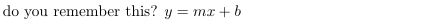Separate from Text

Elements inside the equation or displaymath environments are centered and you cannot use regular line breaks (\\ or a blank line). To write equations across multiple lines, use the environment eqnarray. Compare the two below:

\begin{equation}
y = m x + b
\end{equation}

\begin{eqnarray}
y=mx+b\\
y - b = m x
\end{eqnarray}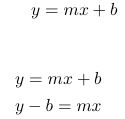Manipulating Fonts Within Math Mode

First let's clarify the distinction between normal text and math symbols that look like fancy letters in the Roman alphabet: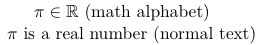If you need to use math alphabet symbols like the first line, you need to use one of the following math alphabet commands:

 Math Alphabet Command Result \mathrm{R}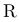\mathtt{R}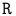\mathbf{R}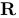\mathsf{R}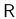\mathit{R}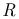\mathcal{R}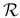\mathbb{R}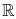When you just want to insert a bit of normal text (as on the second line of the example), you will want to use \mbox{normal text}.

LaTeX does a good job at modifying text sizes to fit the available space in a fraction or other function. But you may want to tweak the size even more. You would then use one of these four commands, all of which are relative to the font size specified in the document class (results are scaled to 160% for greater visibility):

 Size Command Result \displaystyle{\int^1_0 1 dx}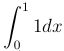\textstyle{\int^1_0 1 dx}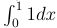\scriptstyle{\int^1_0 1 dx}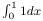\scriptscriptstyle{\int^1_0 1 dx}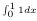Basic Math Symbols

If you are just going to use relatively simple equations, then you will probably find the symbols you need in this chart of basic math symbols on the Introduction to LaTeX page. The documentation also lists LaTeX commands for Greek letters, chemistry and other symbols. For more advanced symbols, see the list here and definitely check out our list of resources.

Elements of a Math Paper

Title and Abstract

To make a title page, you need to specify the title (\title), author (\author) and date (\date) in the preamble, and then instruct LaTeX to make a title page. Go to the general paper documentation for a more in-depth explanation.

You can make an abstract quickly and easily using the abstract environment. The text will be centered, slightly smaller than the normal body text and have bigger margins than the rest of the paper:

\begin{abstract}
The study was a manipulative, growth room experiment that examined the amount (concentration and weight) of iron or copper in eight distinct tissue types and growth variables such as grain yield, weight of individual seeds and the weight of the total grain yield and tissues measured.
\end{abstract}
One independent variables was the time of sampling: anthesis, 18 days after anthesis or at the maturation of grains. The other variable was the amount of minerals given. The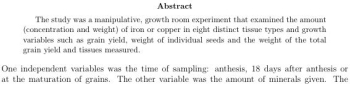Text

If you want to write large blocks of text, you can do so as long as you make sure that you are writing outside of math mode. In that case, you can insert any bits of math you need by bracketing the math with dollar signs.

Graphics and Figures

The easiest way to insert graphics and figures is using GraphicX and \includegraphics, as described on this page. There is also an environment in which you can precisely place lines, letters and simple shapes using only LaTeX markup. The picture environment can be very time consuming to use, and we recommend that you use Adobe Illustrator or other program unless you need to make multiple, very similar and basic images that frequently employ labels. In such a case, the picture environment would come in handy. Books such as A Guide To LaTeX by Kopka and Daly, or Math Into LaTeX by Gratzer, are useful and available from CUS and the library.

Arrays

The environment eqnarray is used to create many lines of equations. You break lines with two backslashes and the lines are numbered automatically. If you do not want each line of calculations to be numbered, use the environment eqnarray*. Equations in eqnarray are centered around the equals sign, whereas equations in multiple equation environments are not. You can see this difference, however subtle, in the following image: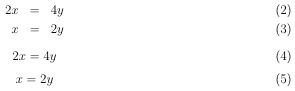Proofs

The proof environment sets up a normal text (not math mode) environment that is automatically titled Proof and ends with the square box symbol for QED.

Tips from Former Math Majors

Spacing for Best Effect

Because math mode ignores spaces, you may wish to force horizontal space. The commands to do so are as follows:

 Horizontal space Result \!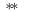\,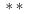\: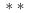\;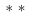Also, stretchy vertical spaces are preferable to the absolute vertical space described in the general paper documentation. Instead, try \bigskip, \medskip and \smallskip.

Physics Papers

While most profs generally frown on lab reports with two columns, physics profs reportedly appreciate the use of REVTeX. This is a document class put out by the American Physical Society and greatly helps with formatting a physics lab report. You can get REVTeX from TUG and learn more about document classes from our Advanced LaTeX page. If you use REVTeX, be sure to read APS's tips.

Hard to Find Symbols

The special symbols section of the Introduction to LaTeX section is limited to very basic symbols. Most (if not all) of the other symbols you need to use will probably be found at the at The Art of Problem Solving. The Comprehensive LaTeX Symbol List has just about every symbol in LaTeX, so definitely check there. It's 91 pages long, so it helps to know the proper name of the symbol or be able to copy-paste the symbol into the search function of your PDF viewer.

A recent math grad would like to point out that there are different epsilons that cannot be interchanged. Luckily, these symbols and others are obtainable using the Greek letters under math mode.

 Input How it appears \epsilon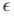\in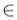\varepsilon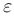\theta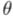\vartheta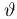\pi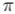\varpi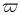\rho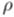\varrho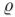\sigma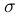\varsigma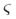Resources

If you have a math-specific problem with LaTeX, you're in luck! This is what LaTeX was built to handle. Most of these resources cover general as well as specific questions and are listed on the Intro to LaTeX page. The fastest and easiest way to look for help with LaTeX is Google.com. Go to Google and include "LaTeX" along with the specific problem or issue.